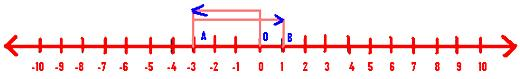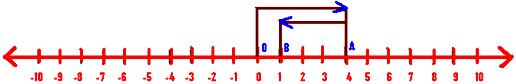# Addition of Integers on a Number Line

Addition of integers on a number line is discussed here. In order to add two integers on a number line, we follow the following step procedure:

### Procedure

#### STEP I:

Draw a number line and mark integers on it.

#### STEP II:

Start from the point representing the first number on the number line.

#### STEP III:

Move as many units as the second number to the

(i) right of the first number, if the second number is positive.

(ii) left of the first number, if the second number is negative.

#### STEP IV:

Obtain the number representing the point reached in the previous step. This number represents the required sum of the given integers.

The following examples will explain the use of the above procedure to add two integers on a number line.

### Represent the following on the number line:

(i) 3 + 4

Solution:

We begin at zero and move 3 units to the right of it to arrive at A representing 3. The second number is positive. So move 4 units to the right to A to arrive at B representing 7.Thus, we have 3 + 4 = 7.

(ii) -3 + 4

Solution:

We begin at zero and first move 3 units to the left of zero to arrive at A which represents -3. The second number is positive 4. So we move 4 units to the right of A to arrive at B representing 1.Thus, we have -3 + 4 = 1.

(iii) 4 + (-3)

Solution:

We begin at zero and first move 4 units to the right of zero to arrive at A which represents 4. The second number is negative 3. So we move 3 units to the left of A to arrive at B representing 1.Thus, we have 4 + (-3) = 1.

(iv) (-3) + (-4)

Solution:

We begin at zero and first move 3 units to the left of zero to arrive at A which represents -3. The second number is negative 4. So we move 4 units to the left of A to arrive at B which represents -7.Thus, we have (-3) + (-4) = -7.

These are the examples of addition of integers on a number line.